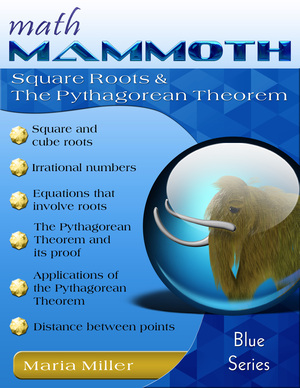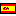GIVEAWAY! Win a Math Mammoth bundle or a grade level of your choice (13 winners!). Take part here. The giveaway runs through Sep 30, 2023.
^
You are here: HomeBlue Series → Square Roots & The Pythagorean Theorem

# Math Mammoth Square Roots & The Pythagorean Theorem77 pages

Sample pages (PDF)
Contents
Square Roots
The Pythagorean Theorem
Applications

## Prices & ordering

Printed copy \$9.305

(credit cards, Google Pay, Paypal, PO)Este libro en español

Math Mammoth Square Roots & The Pythagorean Theorem is a relatively short worktext focusing on irrational numbers, square roots, and the Pythagorean Theorem and its applications.

First, students learn about taking a square root as the opposite operation to squaring a number. They learn about irrational numbers, and how to find approximations to square roots both with a calculator and with a guess-and-check method. Students also practice placing irrational numbers on the number line, using mental math to find their approximate location.

Next, the book has a review lesson on how to convert fractions to decimals. The following lesson has to do with writing decimals as fractions, and teaches a method for converting repeating decimals to fractions.

Then it is time to learn to solve simple equations that involve taking a square or cube root, over the course of two lessons. After learning to solve such equations, students are now fully ready to study the Pythagorean Theorem and apply it.

The Pythagorean Theorem is introduced in the lesson by that name. Students learn to verify that a triangle is a right triangle by checking whether it fulfills the Pythagorean Theorem. They apply their knowledge about square roots and solving equations to solve for an unknown side in a right triangle when two of the sides are given.

Next, students solve a variety of geometric and real-life problems that require the Pythagorean Theorem. This theorem is extremely important in many practical situations. Students should show their work for these word problems to include the equation that results from applying the Pythagorean Theorem to the problem and its solution.

There are literally hundreds of proofs for the Pythagorean Theorem. In this book, we present one easy proof based on geometry (not algebra). As an exercise, students are asked to supply the steps of reasoning to another geometric proof of the theorem. Students also study a proof for the converse of the theorem, which says that if the sides of a triangle fulfill the equation a2 + b2  = c2  then the triangle is a right triangle.

Our last topic is distance between points in the coordinate grid, as this is another simple application of the Pythagorean Theorem.

You can find matching videos for topics in this book at at this link (choose grade 7).

The PDF version of this book can be filled in on a computer, phone, or tablet, using the annotation tools found in many PDF apps. See more.

## Better Yet - Bundle Deals!

*BONUS*: Buy any bundle listed above, and get the fully upgraded Soft-Pak software for FREE (includes 4 math and 2 language arts programs, complete with on screen and printable options).

## How and where to order

You can buy Math Mammoth books at:

• Here at MathMammoth.com website — simply use the "Add to cart" buttons you see on the product pages.
• Rainbow Resource carries printed copies for the Light Blue series books, plus several CDs (Light Blue and Blue series).
• Homeschool Buyers Club offers download versions of the Light Blue series, plus the Blue series bundle.
• Homeschool Planet sells the digital versions of the main curriculum and lesson plans to go with them.
• Lulu sells printed copies for most of the Math Mammoth materials (various series).

By purchasing any of the books, permission IS granted for the teacher (or parent) to reproduce this material to be used with his/her students in a teaching situation; not for commercial resale. However, you are not permitted to share the material with another teacher.

In other words, you are permitted to make copies for the students/children you are teaching, but not for other teachers' usage.

Math Mammoth books are PDF files. I recommend you use Adobe Reader to view them, including if you use a Mac. You can try other PDF viewers, but they may at times either omit or mess up some of the images.
WAIT!

Receive my monthly collection of math tips & resources directly in your inbox — and get a FREE Math Mammoth book!You can unsubscribe at any time.

### Math Mammoth Tour

Confused about the different options? Take a virtual email tour around Math Mammoth! You'll receive:

An initial email to download your GIFT of over 400 free worksheets and sample pages from my books. Six other "TOURSTOP" emails that explain the important things and commonly asked questions concerning Math Mammoth curriculum. (Find out the differences between all these different-colored series!)

This way, you'll have time to digest the information over one or two weeks, plus an opportunity to ask me personally about the curriculum.
A monthly collection of math teaching tips & Math Mammoth updates (unsubscribe any time)### "Mini" Math Teaching Course

This is a little "virtual" 2-week course, where you will receive emails on important topics on teaching math, including:

- How to help a student who is behind
- Troubles with word problems
- Teaching multiplication tables
- Why fractions are so difficult
- The value of mistakes
- Should you use timed tests
- And more!

A GIFT of over 400 free worksheets and sample pages from my books right in the very beginning.A monthly collection of math teaching tips & Math Mammoth updates (unsubscribe any time)
Enter your email to receive math teaching tips, resources, Math Mammoth news & sales, humor, and more! I tend to send out these tips about once monthly, near the beginning of the month, but occasionally you may hear from me twice per month (and sometimes less often).• A GIFT of over 400 free worksheets and sample pages from my books.